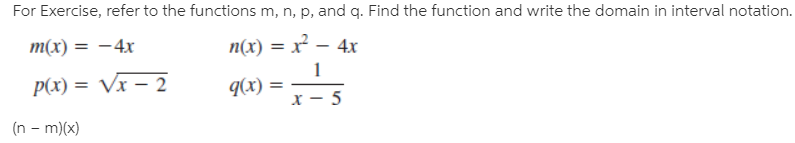# For Exercise, refer to the functions m, n, p, and q. Find the function and write the domain in interval notation. m(x) = –4x n(x) = x² – 4x P(x) = Vx – 2 q(x) = (n - m)(x)

Questionhelp_outlineImage TranscriptioncloseFor Exercise, refer to the functions m, n, p, and q. Find the function and write the domain in interval notation. m(x) = –4x n(x) = x² – 4x P(x) = Vx – 2 q(x) = (n - m)(x) fullscreen